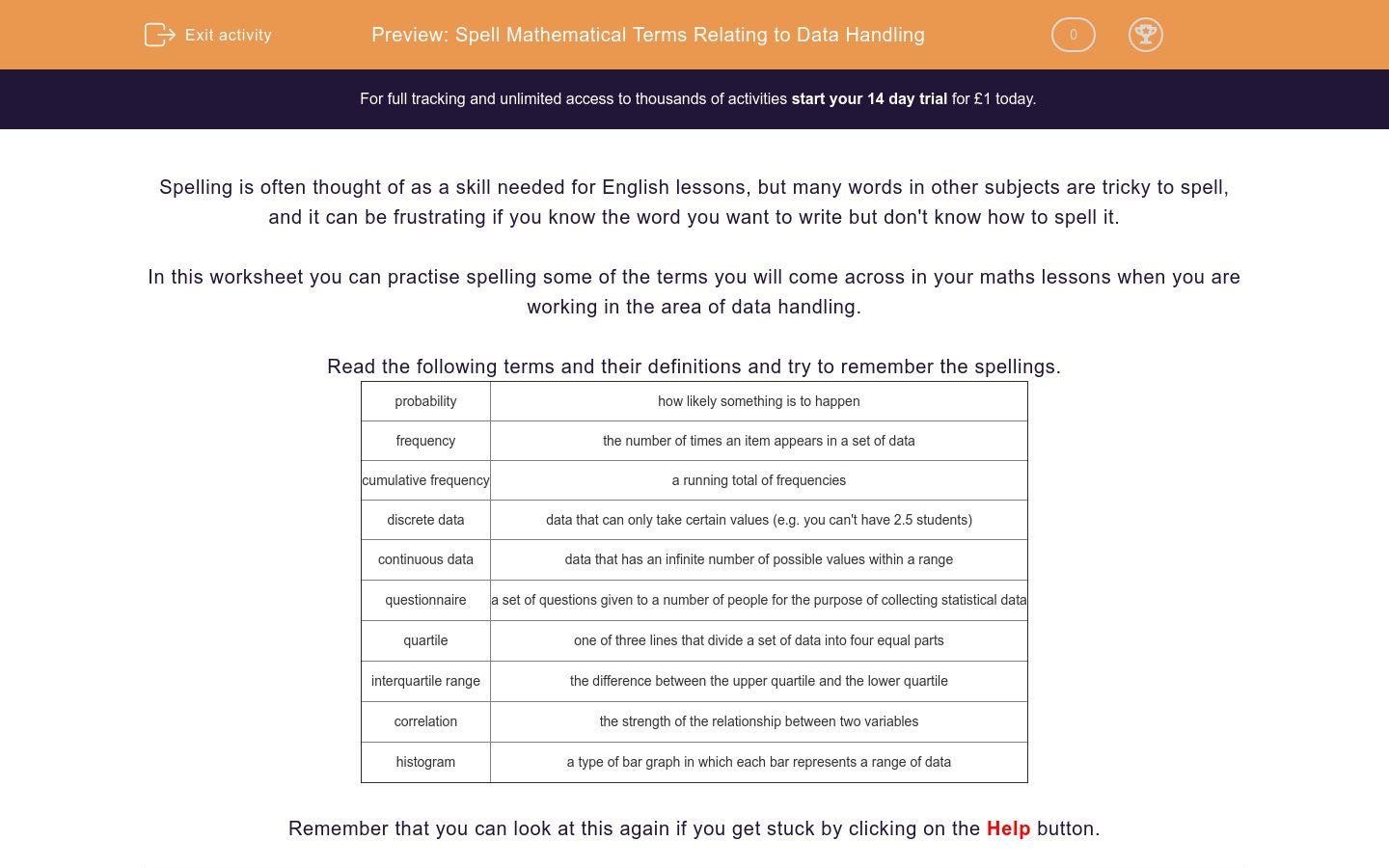# Spell Mathematical Terms Relating to Data Handling

In this worksheet, students practise spelling terms relating to data handling.Key stage:  KS 3

Curriculum topic:   Grammar and Vocabulary

Curriculum subtopic:   Vocabulary and Grammar Awareness

Difficulty level:### QUESTION 1 of 10

Spelling is often thought of as a skill needed for English lessons, but many words in other subjects are tricky to spell, and it can be frustrating if you know the word you want to write but don't know how to spell it.

In this worksheet you can practise spelling some of the terms you will come across in your maths lessons when you are working in the area of data handling.

Read the following terms and their definitions and try to remember the spellings.

 probability how likely something is to happen frequency the number of times an item appears in a set of data cumulative frequency a running total of frequencies discrete data data that can only take certain values (e.g. you can't have 2.5 students) continuous data data that has an infinite number of possible values within a range questionnaire a set of questions given to a number of people for the purpose of collecting statistical data quartile one of three lines that divide a set of data into four equal parts interquartile range the difference between the upper quartile and the lower quartile correlation the strength of the relationship between two variables histogram a type of bar graph in which each bar represents a range of data

Remember that you can look at this again if you get stuck by clicking on the Help button.

a type of bar graph in which each bar represents a range of data

how likely something is to happen

the number of times an item appears in a set of data

data that can only take certain values

data that has an infinite number of possible values within a range

one of three lines that divide a set of data into four equal parts

the strength of the relationship between two variables

a running total of frequencies

a set of questions given to a number of people for the purpose of collecting statistical data

the difference between the upper quartile and the lower quartile

• Question 1

a type of bar graph in which each bar represents a range of data

histogram
EDDIE SAYS
The word ends with a single 'm', not -mme.
• Question 2

how likely something is to happen

probability
EDDIE SAYS
Watch the 'a' in the middle of this one.
• Question 3

the number of times an item appears in a set of data

frequency
EDDIE SAYS
If you can spell 'frequent' then you only need to alter the ending.
• Question 4

data that can only take certain values

discrete
discrete data
EDDIE SAYS
This word is often misspelt because there is another word in English that sounds the same but has a different spelling. 'Discreet' is an adjective that describes the way a person behaves.
• Question 5

data that has an infinite number of possible values within a range

continuous
continuous data
EDDIE SAYS
Be careful with the -uous ending.
• Question 6

one of three lines that divide a set of data into four equal parts

quartile
EDDIE SAYS
If you think of the word 'quarter' it can help with this one.
• Question 7

the strength of the relationship between two variables

correlation
EDDIE SAYS
Remember the double 'r' in this one.
• Question 8

a running total of frequencies

cumulative
cumulative frequency
EDDIE SAYS
There is only one 'm' in 'cumulative'.
• Question 9

a set of questions given to a number of people for the purpose of collecting statistical data

questionnaire
EDDIE SAYS
People often forget the double 'n' in this one.
• Question 10

the difference between the upper quartile and the lower quartile

interquartile range
EDDIE SAYS
If you can spell 'quartile' then this one isn't too tricky.
---- OR ----

Sign up for a £1 trial so you can track and measure your child's progress on this activity.

### What is EdPlace?

We're your National Curriculum aligned online education content provider helping each child succeed in English, maths and science from year 1 to GCSE. With an EdPlace account you’ll be able to track and measure progress, helping each child achieve their best. We build confidence and attainment by personalising each child’s learning at a level that suits them.

Get started